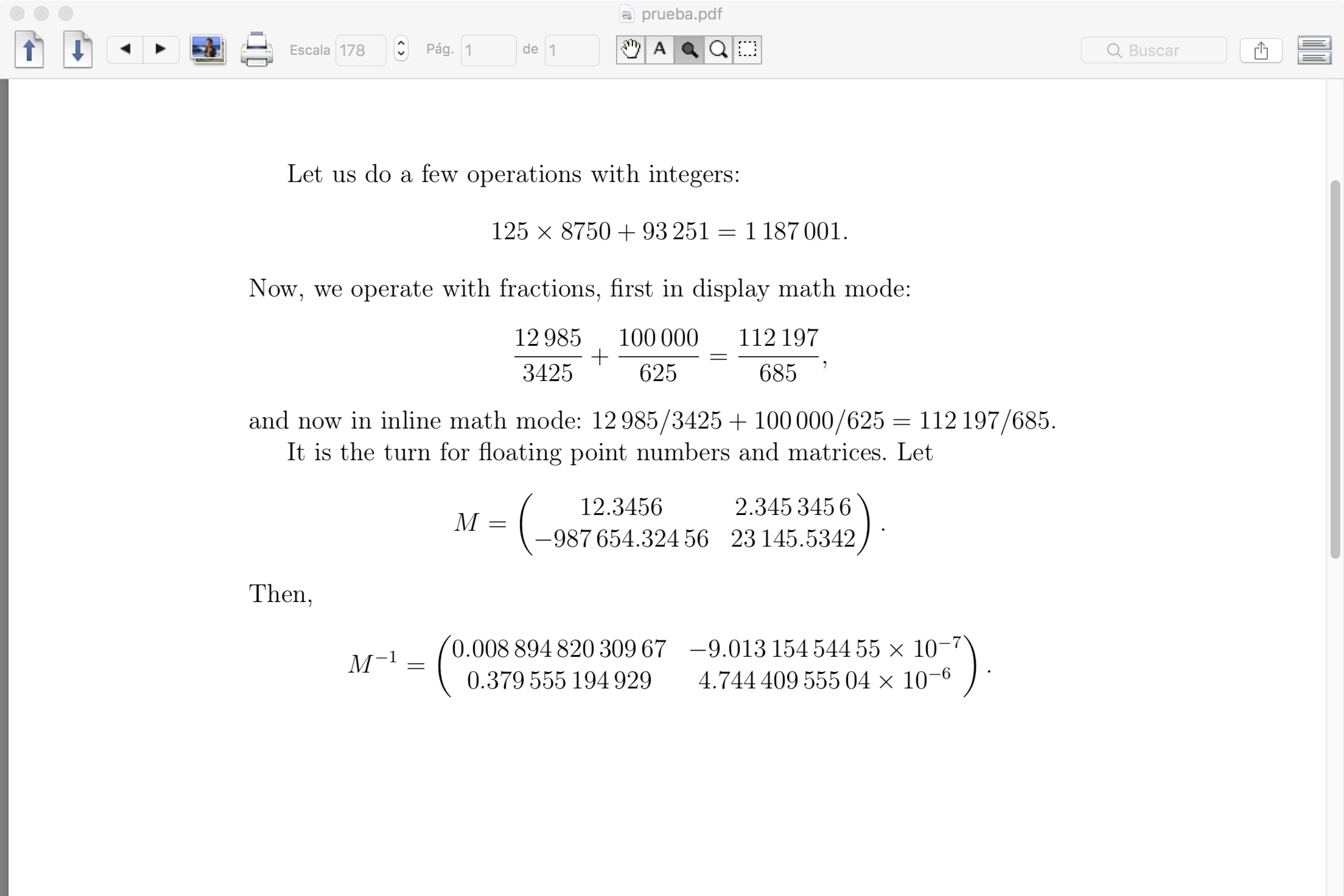# Spanish numbers using LaTeX

Dear SageMath community,

I am currently writing a LaTeX document in Spanish that relies heavily on sageTeX for computations. The rules for writing numbers in Spanish are a little different from English ones. For example, we are prohibited from using commas to separate every 1000 factor, instead using a small space, only if the number is longer than four digits; otherwise, no sign should be used. On the other hand, the decimal point is actually a decimal comma. For example,

• 1,000,000.25 should be written as 1 000 000,25
• 123,456,789.123456789 should be written as 123 456 789,123 456 789
• 4,000 should be written as 4000

Using sageTeX, SageMath's latex command, and LaTeX babel package, it is easy to replace the decimal point with a decimal comma. It is also easy to eliminate the commas for every 1000 factor. However, I haven't been able to put a small space instead.

I could use the siunitx LaTeX package, which has a command, \num, that does exactly this processing. However, it doesn't work with sageTeX's \sage command or any of it's environments. The only solution I can find is to apply the \num command directly to every number generated by SageMath via the latex command.

My problem is: I don't know how to do this, specially in cases where numbers are entries of a matrix, or the coefficients of a polynomial. So, how could I apply the \num command to every number independently using the latex command?

edit retag close merge delete

Sort by » oldest newest most voted

Hello, Sage community. I was able to adapt @Juanjo's answer, but then I did some incantation (which I don't completely understand) that generalized his method. The only problem is that I had to modify the code in the file sage.misc.latex.latex. Here I describe what I did.

First of all, I made a copy of the file I just mentioned, and added a small modification of part of your code. Here is the patch:

@@ -36,6 +36,7 @@
from sage.misc.sage_ostools import have_program
from sage.misc.temporary_file import tmp_dir

@@ -36,6 +36,7 @@
from sage.misc.sage_ostools import have_program
from sage.misc.temporary_file import tmp_dir

+from sage.all import RR, CC
EMBEDDED_MODE = False

@@ -921,6 +922,10 @@
sage: latex((x,2), combine_all=True)
x 2
"""
+        if x in RR:
+            return LatexExpr(r'\num{' + str(x) + '}')
+        elif x in CC:
+            return LatexExpr(r'\num{' + str(x.real_part()) + '+' + str(x.imag_part()) + 'i}')
if has_latex_attr(x):
return LatexExpr(x._latex_())
try:


I saved this as LaTeX.py., then I made an import:

from LaTeX import latex


And here is the part I don't understand:

sage.misc.latex.latex = latex


This last line makes my new latex() function work on matrices, tuples, list, etc. automatically. (Why?)

moreI will provide a parcial answer to the question. Let us consider the following LaTeX code:

\documentclass[12pt]{article}
\usepackage[T1]{fontenc}
\usepackage[utf8]{inputenc}
\usepackage[spanish,es-noshorthands]{babel}
\usepackage{amsmath}
\usepackage{siunitx}
\usepackage{sagetex}

\begin{document}

\begin{sagesilent}
def NumWrap(number):
if number in ZZ or number.parent()==QQ:
return r"\num{"+ str(number) + "}"
else:
return r"\num{"+ str(float(number)) + "}"

def MatWrap(m):
s = r"\begin{pmatrix} "
for nrow in range(m.nrows()):
for ncol in range(m.ncols()-1):
s += NumWrap(m[nrow,ncol]) + " & "
s += NumWrap(m[nrow,-1])
if nrow<m.nrows()-1: s += r" \\ "
s += r" \end{pmatrix}"
return s
\end{sagesilent}

Let us do a few operations with integers:
\begin{equation*}
\num{125}\times\num{8750}+\num{93251} = \sagestr{NumWrap(125*8750+93251)}.
\end{equation*}
Now, we operate with fractions, first in display math mode:
\begin{equation*}
\sisetup{quotient-mode = fraction}
\num{12985/3425} + \num{100000/625}
= \sagestr{NumWrap(12985/3425+100000/625)},
\end{equation*}
and now in inline math mode:
$\num{12985/3425} + \num{100000/625}=\sagestr{NumWrap(12985/3425+100000/625)}$.

It is the turn for floating point numbers and matrices.
\begin{sagesilent}
m = matrix([[12.3456, 2.3453456],[-987654.32456, 23145.5342]])
\end{sagesilent}
Let
\begin{equation*}
M = \sagestr{MatWrap(m)}.
\end{equation*}
Then,
\begin{equation*}
M^{-1} = \sagestr{MatWrap(m.inverse())}.
\end{equation*}

\end{document}


Suppose that x is a number coming from a Sage computation that we want to include in the LaTeX document. The Sage function NumWrap takes a string representation of this number and returns the string \num{str(x)}. This string is passed from Sage to LaTeX by the \sagestr macro, so it can be typeset. For a matrix, MatWrap applies NumWrap to each element and adds LaTeX code to get a pmatrix environment (defined in the amsmath package). I adjoin a screen capture of the document obtained after typesetting the above LaTeX code.more

Hello, @Juanjo. Thank you very much for your answer!!! I had an awful time trying to use the \num LaTeX command through sageTeX. I could find a general solution (see my answer), but I used yours as a starting point. Thanks again!